26 Sep 2019

# Incremental Average and Standard Deviation with Sliding Window

### Moving Average on Streaming Data (4 Part Series)

4. Incremental Average and Standard Deviation with Sliding Window

I was pleasantly surprised recently to get a question from a reader about a couple of my articles, Calculating a Moving Average on Streaming Data and Calculating Standard Deviation on Streaming Data. The question was, instead of updating the statistics cumulatively, would it be possible to consider only a window of fixed size instead?

In other words, say we set the window size to 20 items. Once the window is full, each time a new value comes along, we include it as part of the updated average and standard deviation, but the oldest value is also removed from consideration. Only the most recent 20 items are used (or whatever the window size happens to be).

I thought this was an interesting question, so I decided to try to figure it out. It turns out that we only have to make some small changes to the logic from the earlier articles to make this work. I’ll briefly summarize the derivation and show example code in JavaScript as well.

The diagram below shows the basic idea. We initially have values from x0 to x5 in our window, which has room for 6 items in this case. When we receive a new value, x6, it means we have to remove x0 from the window, since it’s currently the oldest value. As new values come in, we keep sliding the window forward: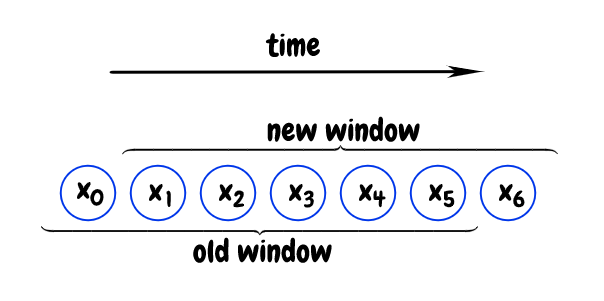## Sliding Average

Let’s start by deriving the moving average within our window, where N corresponds to the window size. The average for values from x1 to xn is as follows: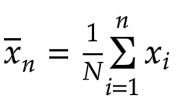It’s basically unchanged from the first article in this series, Calculating a Moving Average on Streaming Data. However, since the size of our window is now fixed, the average up to the previous value, xn-1 is: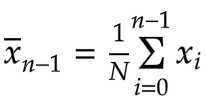Subtracting these two averages, we get the following expression: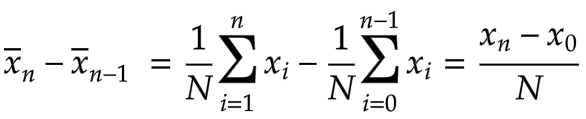The first average consists of a sum of values from x1 to xn. From this, we subtract a sum of values from x0 to xn-1. The only values that don’t cancel each other out are xn and x0. Our final recurrence relation for the incremental average with a sliding window of size N is therefore: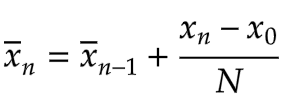That’s all we need to compute the average incrementally with a fixed window size. The corresponding code snippet is below:

``````const meanIncrement = (newValue - poppedValue) / this.count
const newMean = this._mean + meanIncrement
``````

## Sliding Variance and Standard Deviation

Next, let’s derive the relation for d2n.

What is d2? It’s a term I made up for the variance * (n-1), or variance * n, depending on whether we’re talking about sample variance or population variance. For more background on the naming, see my article The Geometry of Standard Deviation.

From Calculating Standard Deviation on Streaming Data, we’ve already derived the following:Again, since our window size remains constant, the equation for d2n-1 has the same form, with the only difference being that it applies to the range of values from x0 to xn-1: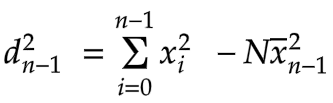When we subtract these two equations, we get:Since the two summations overlap everywhere except at xn and x0, we can simplify this as follows: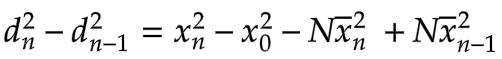We can now factor this expression into the following form:We can also factor the difference of squares on the right: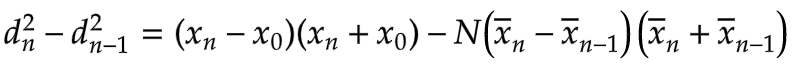Next, we notice that the difference between the current average and the previous average, n - x̄n-1, is (xn - x0)/N, as derived earlier: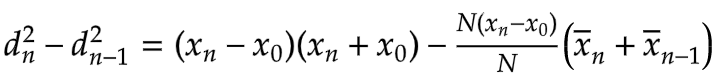We can cancel the N’s to get the following nicely simplified form:To reduce the number of multiplications, we can factor out xn - x0: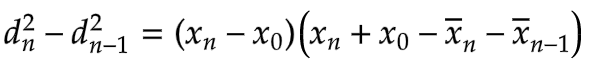Lastly, to get our final recurrence relation, we add d2n-1 to both sides. This gives us the new value of d2 in terms of the previous value and an increment: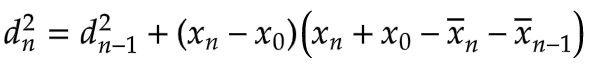The corresponding code is:

``````const dSquaredIncrement = ((newValue - poppedValue)
* (newValue - newMean + poppedValue - this._mean))
const newDSquared = this._dSquared + dSquaredIncrement
``````

## Discussion

We now have a nice way to incrementally calculate the mean, variance, and standard deviation on a sliding window of values. With a cumulative average, which was described in the first article in this series, we have to express the mean in terms of the total number of values received so far - from the very beginning.

That means we will get smaller and smaller fractions as time goes on, which will eventually lead to floating point precision problems. Even more importantly, after a large number of values has come along, a new value will just no longer represent a significant change, regardless of the precision. Here that issue doesn’t come up: Our window size is always the same, and we only need to make adjustments based on the oldest value that is leaving the window, and the new value coming in.

This approach also requires less computation than re-calculating everything in the current window from scratch each time. However, for many real-world applications, I suspect this may not make a huge difference. It should become more useful if the window size is large and the data is streaming in rapidly.

## Code

A demo with full source code for calculating the mean, variance, and standard deviation using a sliding window is available on github:

##nestedsoftware / iterative_stats

### Demo of adjustment to Welford method for calculating mean/variance/stdev that incorporates a sliding window of fixed size over the incoming data

Simple demo that compares two ways to calculate mean/variance/standard deviation over incoming data within a fixed window size. In the first case, we re-calculate the statistics from scratch using all of the values currently within the window. In the second case, we use an adjusted version of Welford's method such that we only need to consider the value entering the window and the oldest value it is replacing.

To run: `node IterativeStatsWithWindow.js`